Like   Tweet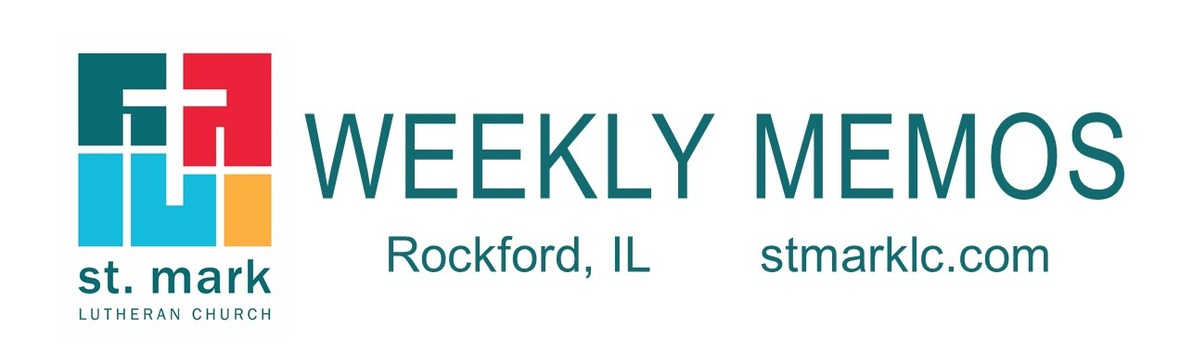table div table+table div table{width:100%;padding:0}table div table+table div table img{width:96.23%;padding:0;float:none}table div table+table div table td{width:100%;padding:0 1.88% 18px}/* styles */## Sunday, July 18

9:00 A.M. Indoor Worship Live-Stream in the Sanctuary
9:00 A.M. Baptism of Claire Noel Iverson
10:00 A.M. Fellowship Hour with Coffee & Donuts
11:00 A.M. Worship on the Lawn
12:30 P.M. VBS Volunteer Training

## Tuesday, July 20

10:00 A.M. Staff Meeting
11:30 A.M. St. Mark Duplicate Bridge - in the cafe
11:30 A.M. Staff Cookout

## Wednesday, July 21

6:30 P.M. Youth Group

## Thursday, July 22

9:00 A.M. Cherry Valley Bridge Group in Cafe
12:30 P.M. - 5:30 P.M. Blood Drive in Fellowship Hall
12:30 P.M. Sew and Sews
4:30 P.M. Kayaking with Pastor Katrina at Rock Cut
6:30 P.M. Emotions Anonymous

## Sunday, July 25

9:00 A.M. Indoor Worship Live-Stream in the Sanctuary
10:00 A.M. Coffee & Donut Hour between services
11:00 A.M. Worship on the Lawn
12:30 P.M. VBS Volunteer Training

 table div table+table+table+table+table div table{width:100%;padding:0}table div table+table+table+table+table div table img{width:96.23%;padding:0;float:none}table div table+table+table+table+table div table td{width:100%;padding:0 1.88% 18px}/* styles */## News and Events

 table div table+table+table+table+table+table+table div table{width:100%;padding:0}table div table+table+table+table+table+table+table div table img{width:96.23%;padding:0;float:none}table div table+table+table+table+table+table+table div table td{width:100%;padding:0 1.88% 18px}/* styles *//* styles */ Join us for Worship Sunday - 8th Sunday after Pentecost 9:00 am - Traditional Worship in the Sanctuary 10:00 am - Fellowship Hour with Coffee & Donuts 11:00 am - Blended Worship on the Lawn- Bring your lawn chair or blanket, or you can stay in your car. Our weekly live-stream will occur during the 9:00 am Sanctuary service. Follow this link for the live-stream service Sunday. While the 9:00 am service will be fully traditional, our 11:00 am service outside will have the same musical feel as all our worship this past year, with a blend of traditional and contemporary music. As always, weather-related changes to our outdoor worship will be announced the evening before.
 table div table+table+table+table+table+table+table+table+table div table{width:100%;padding:0}table div table+table+table+table+table+table+table+table+table div table img{width:96.23%;padding:0;float:none}table div table+table+table+table+table+table+table+table+table div table td{width:100%;padding:0 1.88% 18px}/* styles *//* styles */ Blood Drive St. Mark will be sponsoring a blood drive on Thursday, July 22 from 12:30 - 5:30 P.M. You can schedule a time by calling Jill Davenport at 815-398-3557 or by clicking on this link.The actual blood donation usually takes less than ten minutes. The entire process from the time you sign in to the time you leave takes about an hour. There are four steps to donating blood: medical history, mini-physical, donation and snacks. All blood types needed!
 table div table+table+table+table+table+table+table+table+table+table+table+table div table{width:100%;padding:0}table div table+table+table+table+table+table+table+table+table+table+table+table div table img{width:96.23%;padding:0;float:none}table div table+table+table+table+table+table+table+table+table+table+table+table div table td{width:100%;padding:0 1.88% 18px}/* styles */Worship on the Lawn
Join us on the lawn by the playground every Sunday at 11:00 for worship. Grab your lawn chair, blanket, and your family as we worship together. Let's get outside to enjoy St. Mark's beautiful outdoors.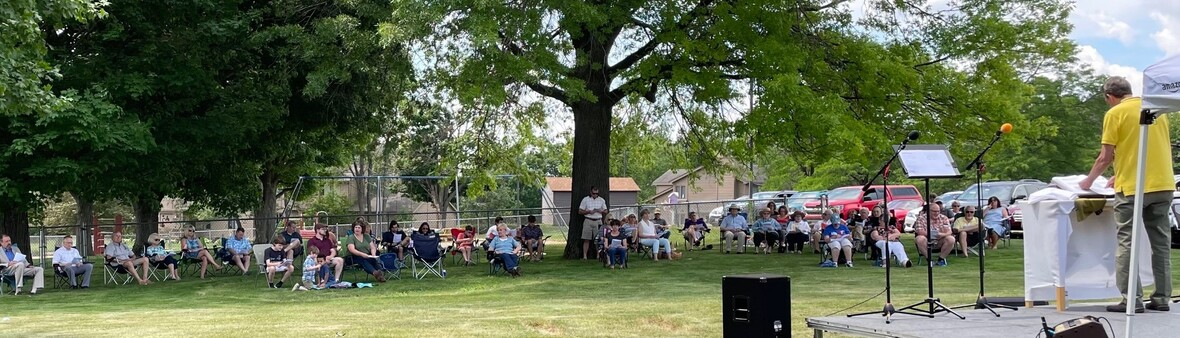table div table+table+table+table+table+table+table+table+table+table+table+table+table+table+table div table{width:100%;padding:0}table div table+table+table+table+table+table+table+table+table+table+table+table+table+table+table div table img{width:96.23%;padding:0;float:none}table div table+table+table+table+table+table+table+table+table+table+table+table+table+table+table div table td{width:100%;padding:0 1.88% 18px}/* styles */Coffee and Donuts are Back!
Our fellowship hour will be between our two worship services from 10:00 - 11:00 a.m. outside under the canopy at the south doors. Libby Malenchik is our hospitality host and will be ready to see you Sunday. Come for a donut, coffee and conversation outside or you may find a table in the cafe.

 table div table+table+table+table+table+table+table+table+table+table+table+table+table+table+table+table+table div table{width:100%;padding:0}table div table+table+table+table+table+table+table+table+table+table+table+table+table+table+table+table+table div table img{width:96.23%;padding:0;float:none}table div table+table+table+table+table+table+table+table+table+table+table+table+table+table+table+table+table div table td{width:100%;padding:0 1.88% 18px}/* styles */table div table+table+table+table+table+table+table+table+table+table+table+table+table+table+table+table+table+table+table div table{width:100%;padding:0}table div table+table+table+table+table+table+table+table+table+table+table+table+table+table+table+table+table+table+table div table img{width:96.23%;padding:0;float:none}table div table+table+table+table+table+table+table+table+table+table+table+table+table+table+table+table+table+table+table div table td{width:100%;padding:0 1.88% 18px}/* styles */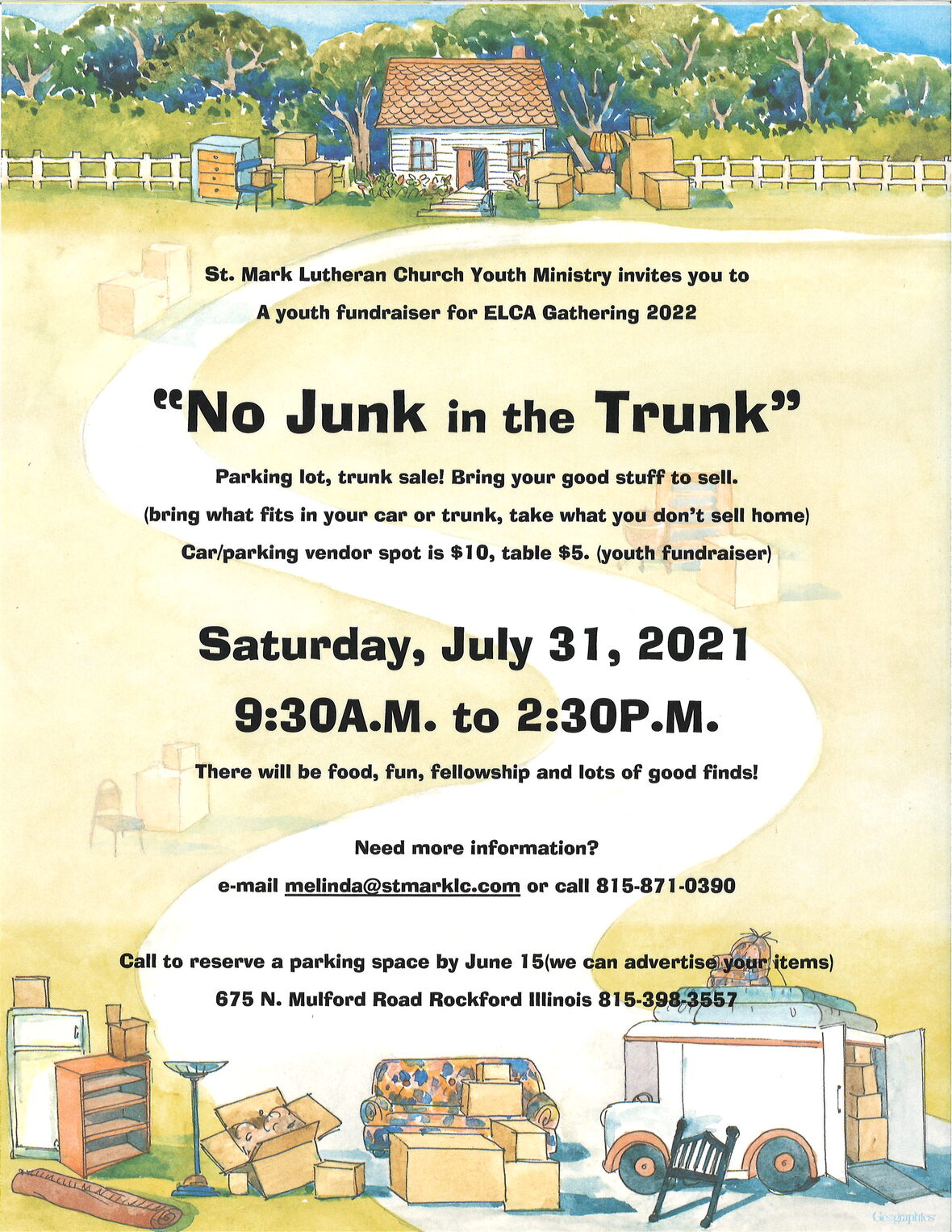If you are on the “fence,” the time is now to sign-up for the parking lot sale.
We will have a great variety of items. Come meet, shop, and enjoy walking around the St. Mark grounds. There will be golf clubs, putting green, steam iron, a wok, dust buster, exercise equipment, chair massage cushion, children’s books and cd’s, and a relish tray and so much more! Invite your friends and family!

 table div table+table+table+table+table+table+table+table+table+table+table+table+table+table+table+table+table+table+table+table+table+table div table{width:100%;padding:0}table div table+table+table+table+table+table+table+table+table+table+table+table+table+table+table+table+table+table+table+table+table+table div table img{width:96.23%;padding:0;float:none}table div table+table+table+table+table+table+table+table+table+table+table+table+table+table+table+table+table+table+table+table+table+table div table td{width:100%;padding:0 1.88% 18px}/* styles */Grand Piano Fund Drive
The St. Mark Music program has a unique opportunity to purchase a second grand piano to support our wonderful music ministry. We recently found a used Yamaha grand piano at a great price, that is identical to our current grand piano. We would like to have a grand piano positioned most of the time in Fellowship Hall, to support our 11:00 second service. We also want to use this second grand piano to support special music events at both Christmas and Easter, along with other concert events and programs.

The total cost to St. Mark to purchase is \$6,500. This includes moving it from Janesville, purchase a rolling cradle, and tuning the piano. The Worship & Music committee can fund a portion of this but we need the support of the congregation. Can you help? Send in your check or use our online payment link below and help us secure this instrument. If paying through the link under the FUND select Grand Piano Fund Drive. Thank you.

 table div table+table+table+table+table+table+table+table+table+table+table+table+table+table+table+table+table+table+table+table+table+table+table+table+table div table{width:100%;padding:0}table div table+table+table+table+table+table+table+table+table+table+table+table+table+table+table+table+table+table+table+table+table+table+table+table+table div table img{width:96.23%;padding:0;float:none}table div table+table+table+table+table+table+table+table+table+table+table+table+table+table+table+table+table+table+table+table+table+table+table+table+table div table td{width:100%;padding:0 1.88% 18px}/* styles */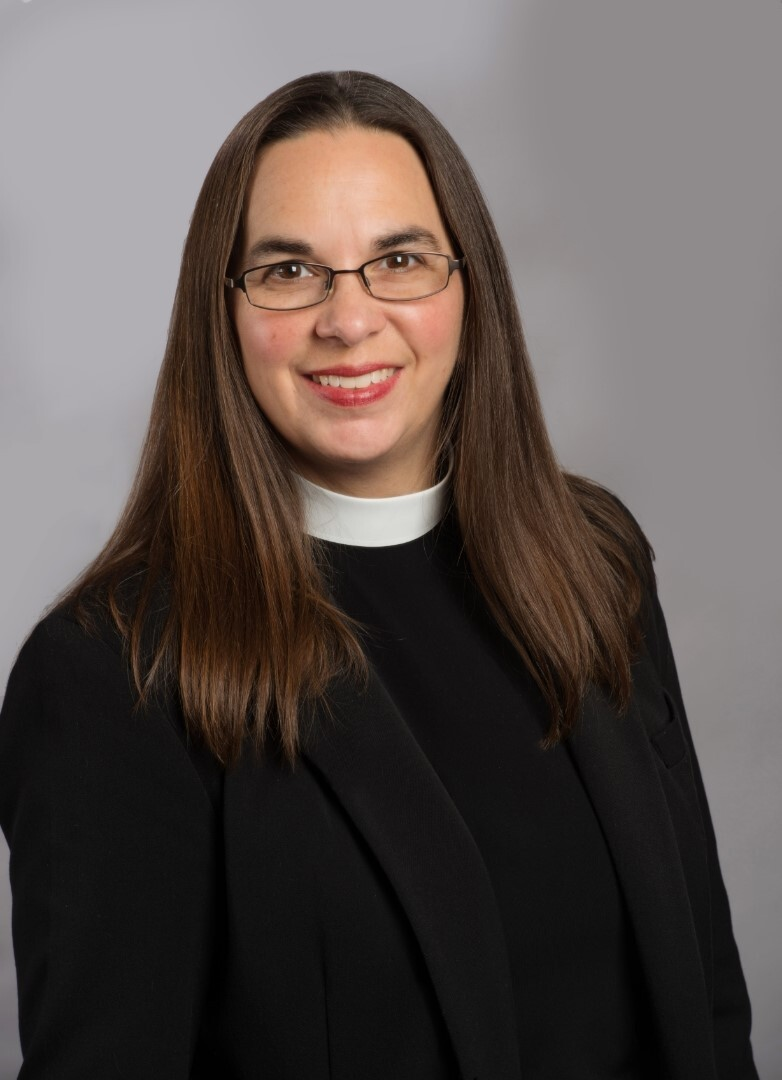Meet with Pastor Katrina
Would you like a chance to chat with me? If so, I have blocked out 45-minute time slots on Wednesday and Thursday afternoons in August for folks to meet with me at St. Mark. Please call the church office at (815)398-3557 between the hours of 9:00 am and 3:00 pm to schedule a time to meet with me. I look forward to meeting you and getting to know you all! Peace, Pastor Katrina

 table div table+table+table+table+table+table+table+table+table+table+table+table+table+table+table+table+table+table+table+table+table+table+table+table+table+table+table div table{width:100%;padding:0}table div table+table+table+table+table+table+table+table+table+table+table+table+table+table+table+table+table+table+table+table+table+table+table+table+table+table+table div table img{width:96.23%;padding:0;float:none}table div table+table+table+table+table+table+table+table+table+table+table+table+table+table+table+table+table+table+table+table+table+table+table+table+table+table+table div table td{width:100%;padding:0 1.88% 18px}/* styles */Sign up for Altar Flowers There are some dates still available to sponsor a flower arrangement on Sundays. This can be in honor or memory of someone or just because. The price for one bouquet is \$35.

To sign up for flowers you can call the office at 815-398-3557, or click on this link. If you sign up online, put your memory or honor of in the comment line and pay here or write a check to St. Mark.

If you have the mobile app you can do all of it on there.
You may take your flowers home after the service or stop by the office during the week to pick them up.

 table div table+table+table+table+table+table+table+table+table+table+table+table+table+table+table+table+table+table+table+table+table+table+table+table+table+table+table+table+table div table{width:100%;padding:0}table div table+table+table+table+table+table+table+table+table+table+table+table+table+table+table+table+table+table+table+table+table+table+table+table+table+table+table+table+table div table img{width:96.23%;padding:0;float:none}table div table+table+table+table+table+table+table+table+table+table+table+table+table+table+table+table+table+table+table+table+table+table+table+table+table+table+table+table+table div table td{width:100%;padding:0 1.88% 18px}/* styles */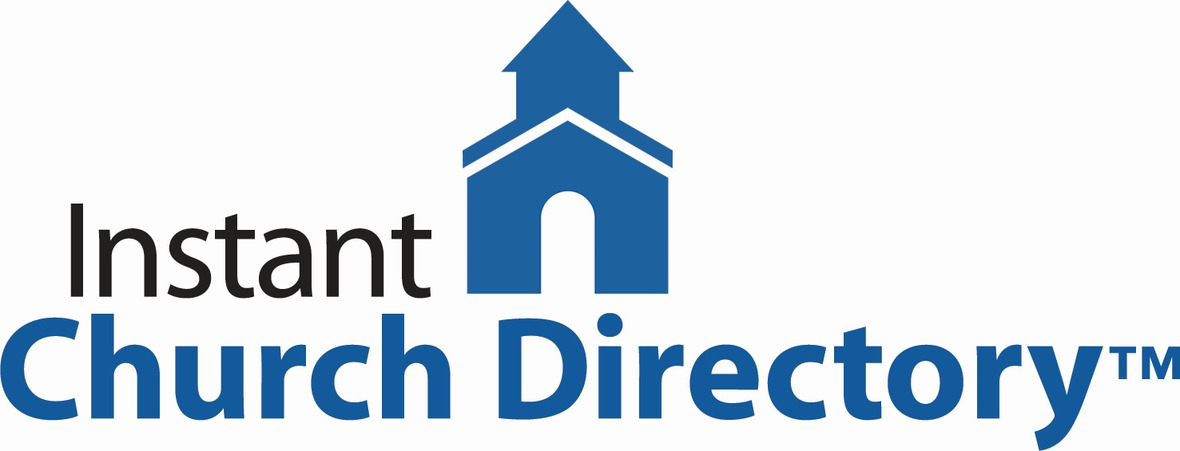By now a lot of us have the church directory app or an online account and love it!

If you haven't gotten around to it, take a few minutes to follow the instructions below. You don't need to have a smartphone to have the directory, you can create an account on your computer and use the directory online. It is simple and easy to use. We do have paper copies available for you if you don't have access to a computer, just call the office to let us know.

To submit a photo for the directory follow the link below to easily upload your own photo to insert into the church directory. On your phone? Snap a quick photo of you or your family and upload it. If you want to stop by the church office, we can take the picture for you.

Instant Church Directory

Instant Church Directory is a simple way to view your Church Directory. Never deal with an outdated directory again, any changes made by church staff are immediately synced to the app.

Mobile App Login Instructions for Android & Kindle Devices

We also have a form for you to complete to make sure we have the most current information for the directory. There are paper
forms at church or click on the link to Church Directory Form

If you need any assistance or have questions, please call Jill Davenport at 815-398-3557 or stop by the office.

 table div table+table+table+table+table+table+table+table+table+table+table+table+table+table+table+table+table+table+table+table+table+table+table+table+table+table+table+table+table+table+table div table{width:100%;padding:0}table div table+table+table+table+table+table+table+table+table+table+table+table+table+table+table+table+table+table+table+table+table+table+table+table+table+table+table+table+table+table+table div table img{width:96.23%;padding:0;float:none}table div table+table+table+table+table+table+table+table+table+table+table+table+table+table+table+table+table+table+table+table+table+table+table+table+table+table+table+table+table+table+table div table td{width:100%;padding:0 1.88% 18px}/* styles */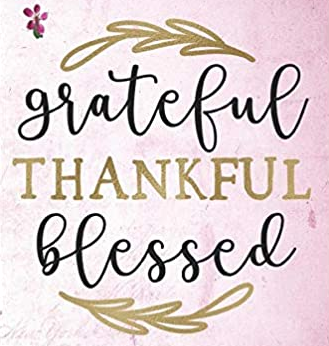In one of our daily devotions, there was a story about God creating birds with wings. However, the wings were so heavy the birds could not fly. The burden of the heavy wings kept the birds on the ground. Soon the burdens from the wings made the birds stronger and eventually they were able to fly. The birds’ burdens turned into blessings.

Burdens make us stronger. Kevin and I are carrying some heavy burdens now. But The Lord has been giving us strength all along the way. And our wonderful St Mark family is right there with us too. Our St Mark family is such a blessing to us.

We want to thank everyone for their prayers and the beautiful cards we have received. We will be much stronger on the other side with God and all our friends by our side.
God’s Peace!
Kevin and Jan Cotter

 table div table+table+table+table+table+table+table+table+table+table+table+table+table+table+table+table+table+table+table+table+table+table+table+table+table+table+table+table+table+table+table+table+table div table{width:100%;padding:0}table div table+table+table+table+table+table+table+table+table+table+table+table+table+table+table+table+table+table+table+table+table+table+table+table+table+table+table+table+table+table+table+table+table div table img{width:96.23%;padding:0;float:none}table div table+table+table+table+table+table+table+table+table+table+table+table+table+table+table+table+table+table+table+table+table+table+table+table+table+table+table+table+table+table+table+table+table div table td{width:100%;padding:0 1.88% 18px}/* styles */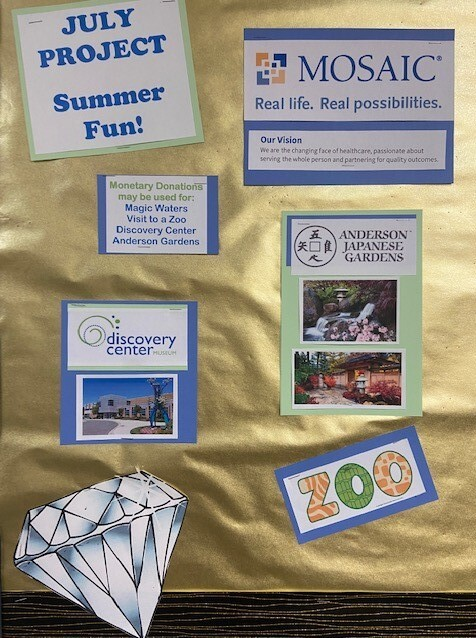/* styles */ Celebrating 60 years of St. Mark Church July collection is gathering items for Mosaic Group Homes for adults with intellectual disabilities. Through the pandemic, the residents were extremely isolated. There are eight Mosaic homes in Rockford that house 60 residents. We are gathering donations of event tickets,(such as Discovery Center, the zoo, etc) chalk, bubbles, and yard games such as “corn hole.” If you are a woodworker we are especially looking for people to make sets of “corn hole” games. We will get the bean bags that go with the game. Monetary donations can be donated on the church app, stmarklc.com or by check to the office. We can really make a difference in the life of some very special people. IF you would like to know more please e-mail or call Melinda Alekna 815-871-0390, melinda@stmarklc.com
 table div table+table+table+table+table+table+table+table+table+table+table+table+table+table+table+table+table+table+table+table+table+table+table+table+table+table+table+table+table+table+table+table+table+table+table+table div table{width:100%;padding:0}table div table+table+table+table+table+table+table+table+table+table+table+table+table+table+table+table+table+table+table+table+table+table+table+table+table+table+table+table+table+table+table+table+table+table+table+table div table img{width:96.23%;padding:0;float:none}table div table+table+table+table+table+table+table+table+table+table+table+table+table+table+table+table+table+table+table+table+table+table+table+table+table+table+table+table+table+table+table+table+table+table+table+table div table td{width:100%;padding:0 1.88% 18px}/* styles *//* styles */ Katie’s Cup – coffee, conversation and community at 502 7th St. Every Thursday Morning 9:00 - 1:00 - Midtown Farmers Market with Music Every Thursday 9:15 Walking Club July 17 at 6 pm - Outdoor concert by Truman Ridge July 20, 11 am - Katie’s Cup Conversation on Zoom. Pr. White-Newgrem from Second First Church on “Bloom Where You are Planted,” outreach in Rockford
 table div table+table+table+table+table+table+table+table+table+table+table+table+table+table+table+table+table+table+table+table+table+table+table+table+table+table+table+table+table+table+table+table+table+table+table+table+table+table div table{width:100%;padding:0}table div table+table+table+table+table+table+table+table+table+table+table+table+table+table+table+table+table+table+table+table+table+table+table+table+table+table+table+table+table+table+table+table+table+table+table+table+table+table div table img{width:96.23%;padding:0;float:none}table div table+table+table+table+table+table+table+table+table+table+table+table+table+table+table+table+table+table+table+table+table+table+table+table+table+table+table+table+table+table+table+table+table+table+table+table+table+table div table td{width:100%;padding:0 1.88% 18px}/* styles */SAVE THE DATE
Friday - Sunday, Sept. 10-12.
A weekend filled with fun, community, celebration, music and concerts, and worship. More details coming soon... You won't want to miss the fun!table div table+table+table+table+table+table+table+table+table+table+table+table+table+table+table+table+table+table+table+table+table+table+table+table+table+table+table+table+table+table+table+table+table+table+table+table+table+table+table+table+table div table{width:100%;padding:0}table div table+table+table+table+table+table+table+table+table+table+table+table+table+table+table+table+table+table+table+table+table+table+table+table+table+table+table+table+table+table+table+table+table+table+table+table+table+table+table+table+table div table img{width:96.23%;padding:0;float:none}table div table+table+table+table+table+table+table+table+table+table+table+table+table+table+table+table+table+table+table+table+table+table+table+table+table+table+table+table+table+table+table+table+table+table+table+table+table+table+table+table+table div table td{width:100%;padding:0 1.88% 18px}/* styles */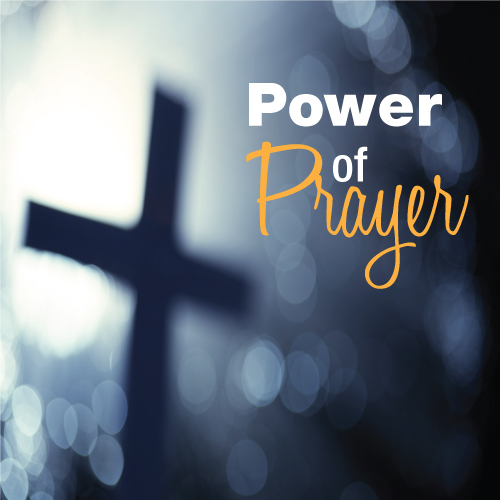St. Mark Prayer Chain
Would you like to be a part of the St. Mark prayer chain? Prayer requests come into the church office weekly. As we receive prayer requests we would send out an e-mail and ask that you pray daily for the persons mentioned in the requests. All requests are to remain confidential. Please e-mail melinda@stmarklc.com if you would like to participate in the prayer chain. “Be anxious for nothing, but in everything by prayer and supplication, with thanksgiving, let your requests be made known to God.” Colossians 4:2.

 table div table+table+table+table+table+table+table+table+table+table+table+table+table+table+table+table+table+table+table+table+table+table+table+table+table+table+table+table+table+table+table+table+table+table+table+table+table+table+table+table+table+table+table div table{width:100%;padding:0}table div table+table+table+table+table+table+table+table+table+table+table+table+table+table+table+table+table+table+table+table+table+table+table+table+table+table+table+table+table+table+table+table+table+table+table+table+table+table+table+table+table+table+table div table img{width:96.23%;padding:0;float:none}table div table+table+table+table+table+table+table+table+table+table+table+table+table+table+table+table+table+table+table+table+table+table+table+table+table+table+table+table+table+table+table+table+table+table+table+table+table+table+table+table+table+table+table div table td{width:100%;padding:0 1.88% 18px}/* styles */## Youth Ministry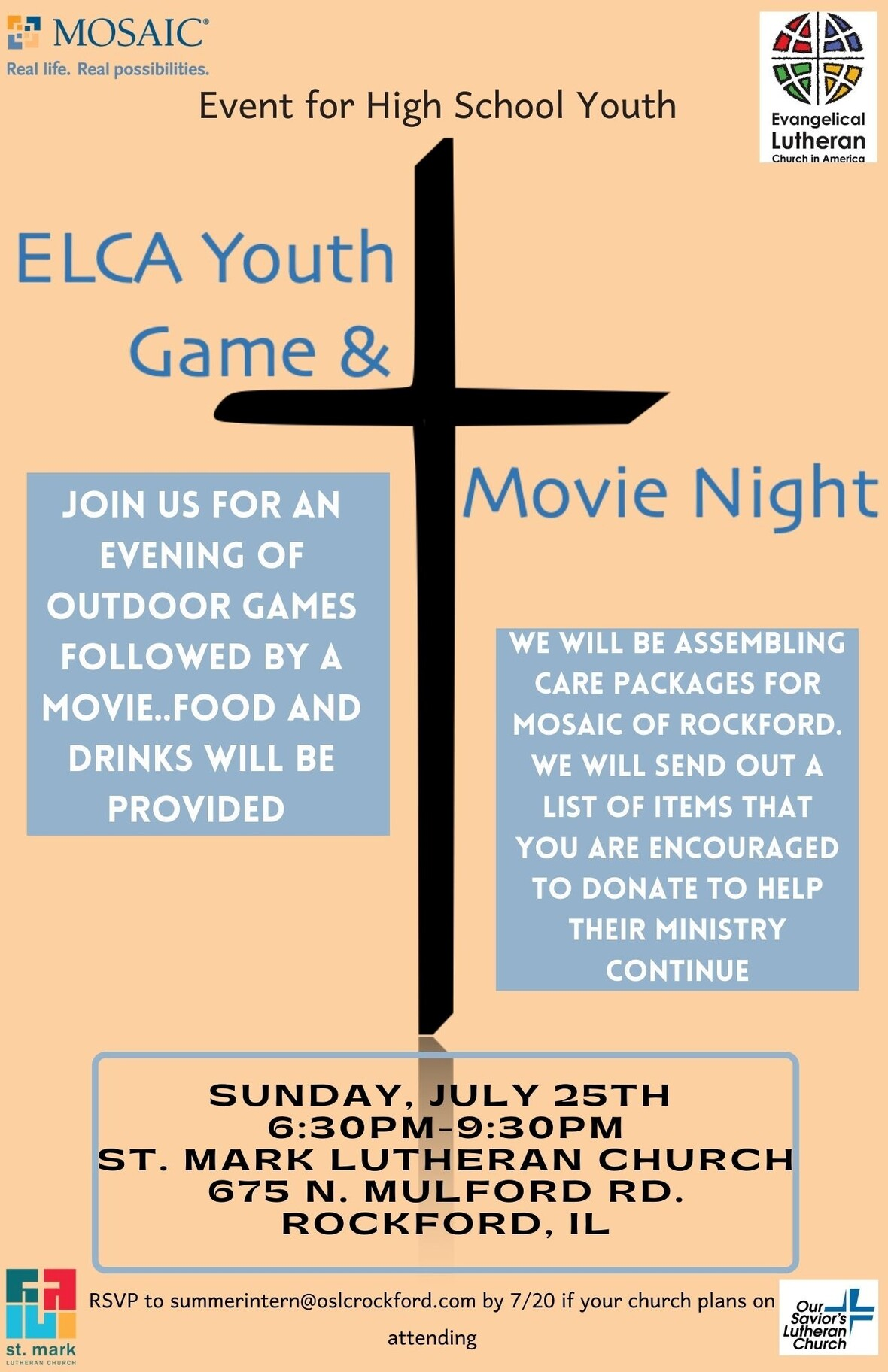table div table+table+table+table+table+table+table+table+table+table+table+table+table+table+table+table+table+table+table+table+table+table+table+table+table+table+table+table+table+table+table+table+table+table+table+table+table+table+table+table+table+table+table+table+table+table+table div table{width:100%;padding:0}table div table+table+table+table+table+table+table+table+table+table+table+table+table+table+table+table+table+table+table+table+table+table+table+table+table+table+table+table+table+table+table+table+table+table+table+table+table+table+table+table+table+table+table+table+table+table+table div table img{width:96.23%;padding:0;float:none}table div table+table+table+table+table+table+table+table+table+table+table+table+table+table+table+table+table+table+table+table+table+table+table+table+table+table+table+table+table+table+table+table+table+table+table+table+table+table+table+table+table+table+table+table+table+table+table div table td{width:100%;padding:0 1.88% 18px}/* styles */Parents and Youth who are entering 8th grade through high school…
Boundless…God Beyond Measure. ELCA Youth Gathering 2022
Our youth typically have one opportunity to attend the Youth Gathering. Summer 2022 is the next opportunity for our young people to attend. Think about it, pray about it. Discounted early sign-up starts in September. We want each of our high school youth to have the ability to attend. Join us at the Gathering 2022 in Minneapolis, Minnesota!

Please e-mail melinda@stmarklc.com or call 815-871-0390 with any questions.

 table div table+table+table+table+table+table+table+table+table+table+table+table+table+table+table+table+table+table+table+table+table+table+table+table+table+table+table+table+table+table+table+table+table+table+table+table+table+table+table+table+table+table+table+table+table+table+table+table+table div table{width:100%;padding:0}table div table+table+table+table+table+table+table+table+table+table+table+table+table+table+table+table+table+table+table+table+table+table+table+table+table+table+table+table+table+table+table+table+table+table+table+table+table+table+table+table+table+table+table+table+table+table+table+table+table div table img{width:96.23%;padding:0;float:none}table div table+table+table+table+table+table+table+table+table+table+table+table+table+table+table+table+table+table+table+table+table+table+table+table+table+table+table+table+table+table+table+table+table+table+table+table+table+table+table+table+table+table+table+table+table+table+table+table+table div table td{width:100%;padding:0 1.88% 18px}/* styles */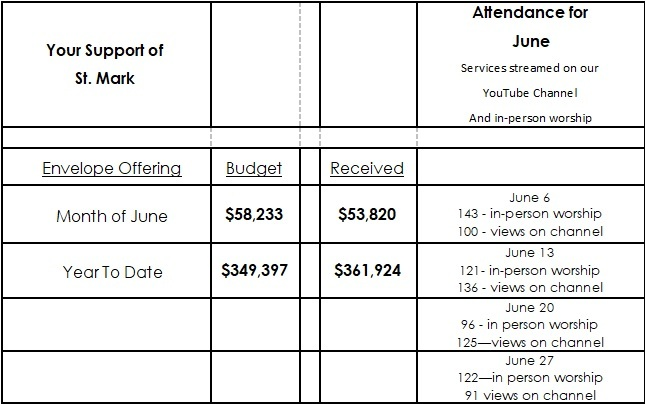table div table+table+table+table+table+table+table+table+table+table+table+table+table+table+table+table+table+table+table+table+table+table+table+table+table+table+table+table+table+table+table+table+table+table+table+table+table+table+table+table+table+table+table+table+table+table+table+table+table+table+table div table{width:100%;padding:0}table div table+table+table+table+table+table+table+table+table+table+table+table+table+table+table+table+table+table+table+table+table+table+table+table+table+table+table+table+table+table+table+table+table+table+table+table+table+table+table+table+table+table+table+table+table+table+table+table+table+table+table div table img{width:96.23%;padding:0;float:none}table div table+table+table+table+table+table+table+table+table+table+table+table+table+table+table+table+table+table+table+table+table+table+table+table+table+table+table+table+table+table+table+table+table+table+table+table+table+table+table+table+table+table+table+table+table+table+table+table+table+table+table div table td{width:100%;padding:0 1.88% 18px}/* styles */## Our St. Mark members:

Dennis Clark, Linda Vivian, Tim Panjkovich, Jack Sowl, Jan Stringer, Lorine Kortus, Jan Cotter, Roger Lind, Diane Swanson, Sherri Lindquist, Bette Patterson, Keith Haggestad.

## Family and friends of St. Mark members:

Clyde Webster, Bob Pollock, Ezra Mark Wiegel, Ed Benfield, Conrad Semmelroth, Nici King, Vicki Kalnins, Kate Cuccaro, Debbie Adams, Wanda Adamson, Milane Bagel, Janet Webster, Rae Ann McIntire, Linda Johnson, Eva Love Sherbondy, Norma Comstock, Carolyn King, Ashton Henley.

## Those serving in the military and their families:

Hunter Haggestad, Tristan Davenport, Scot Hornick,
Bailey Panjkovich, Xander Gehrke, Jim Tammen, Brandon Ballenger, Taylor Eversole, Dean Barron.

Strengthen and encourage those in public health services and in the medical profession: care-givers, nurses, attendants, doctors, all who commit themselves to care for the sick and their families.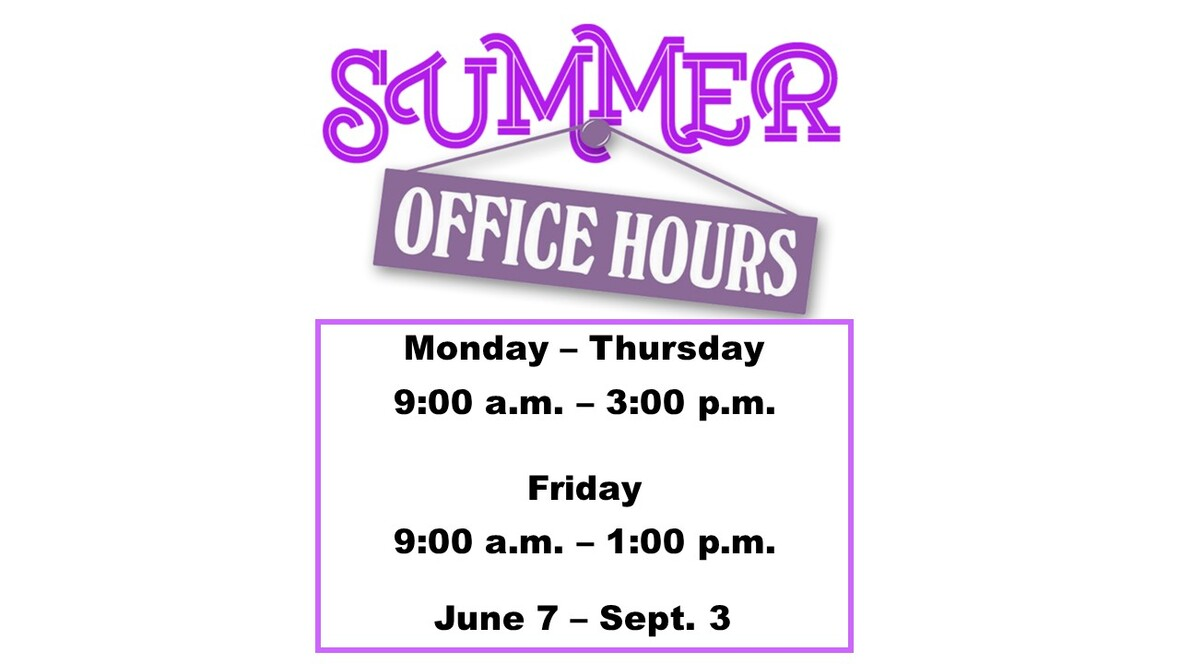table div table+table+table+table+table+table+table+table+table+table+table+table+table+table+table+table+table+table+table+table+table+table+table+table+table+table+table+table+table+table+table+table+table+table+table+table+table+table+table+table+table+table+table+table+table+table+table+table+table+table+table+table+table+table+table div table{width:100%;padding:0}table div table+table+table+table+table+table+table+table+table+table+table+table+table+table+table+table+table+table+table+table+table+table+table+table+table+table+table+table+table+table+table+table+table+table+table+table+table+table+table+table+table+table+table+table+table+table+table+table+table+table+table+table+table+table+table div table img{width:96.23%;padding:0;float:none}table div table+table+table+table+table+table+table+table+table+table+table+table+table+table+table+table+table+table+table+table+table+table+table+table+table+table+table+table+table+table+table+table+table+table+table+table+table+table+table+table+table+table+table+table+table+table+table+table+table+table+table+table+table+table+table div table td{width:100%;padding:0 1.88% 18px}/* styles *//* styles */ Summer Office Hours Monday through Thursday 9:00 A.M. - 3:00 P.M. Friday 9:00 A.M. - 1:00 P.M. Telephone: 815-398-3557 Publications St. Mark Memos, the weekly e-newsletter, is distributed each Thursday. The deadline for submission is Monday by 3:00 P.M. Please click to send submissions. Worship Services Miss a Sunday at St. Mark? Please visit our website and watch the service online. Please click to watch.
 table div table+table+table+table+table+table+table+table+table+table+table+table+table+table+table+table+table+table+table+table+table+table+table+table+table+table+table+table+table+table+table+table+table+table+table+table+table+table+table+table+table+table+table+table+table+table+table+table+table+table+table+table+table+table+table+table+table div table{width:100%;padding:0}table div table+table+table+table+table+table+table+table+table+table+table+table+table+table+table+table+table+table+table+table+table+table+table+table+table+table+table+table+table+table+table+table+table+table+table+table+table+table+table+table+table+table+table+table+table+table+table+table+table+table+table+table+table+table+table+table+table div table img{width:96.23%;padding:0;float:none}table div table+table+table+table+table+table+table+table+table+table+table+table+table+table+table+table+table+table+table+table+table+table+table+table+table+table+table+table+table+table+table+table+table+table+table+table+table+table+table+table+table+table+table+table+table+table+table+table+table+table+table+table+table+table+table+table+table div table td{width:100%;padding:0 1.88% 18px}/* styles */